Miscellaneous

Chapter 1 Class 11 Sets (Term 1)
Serial order wise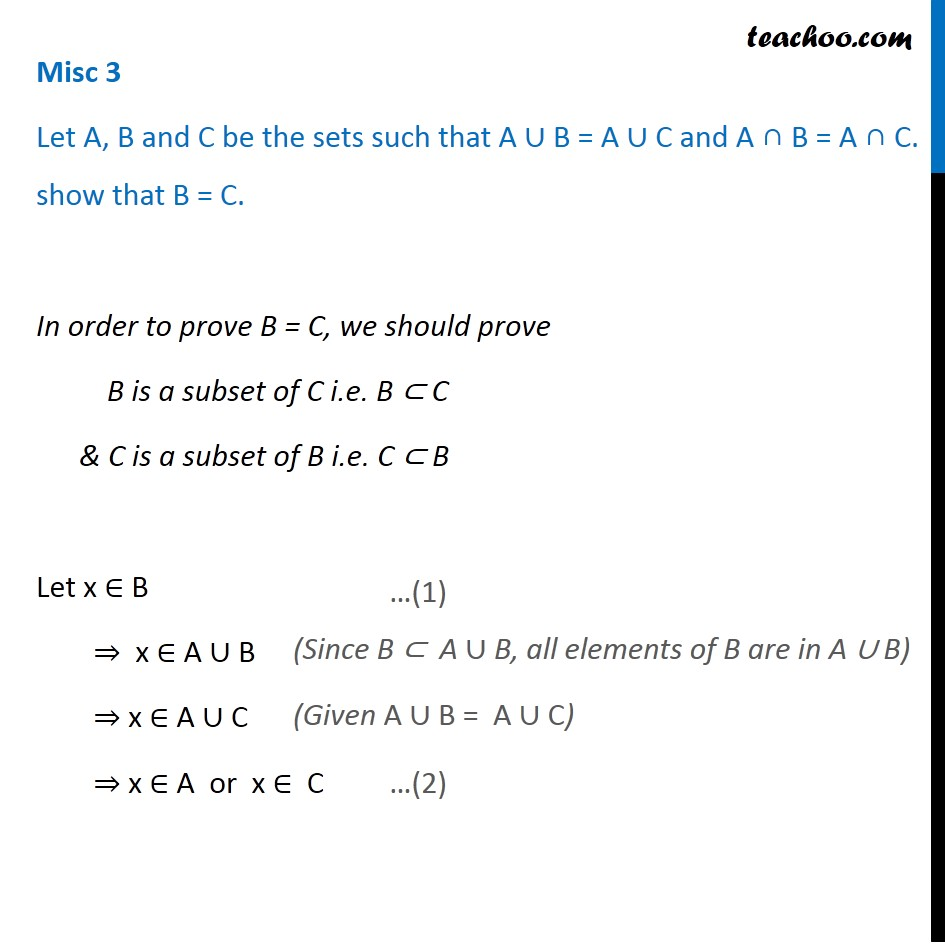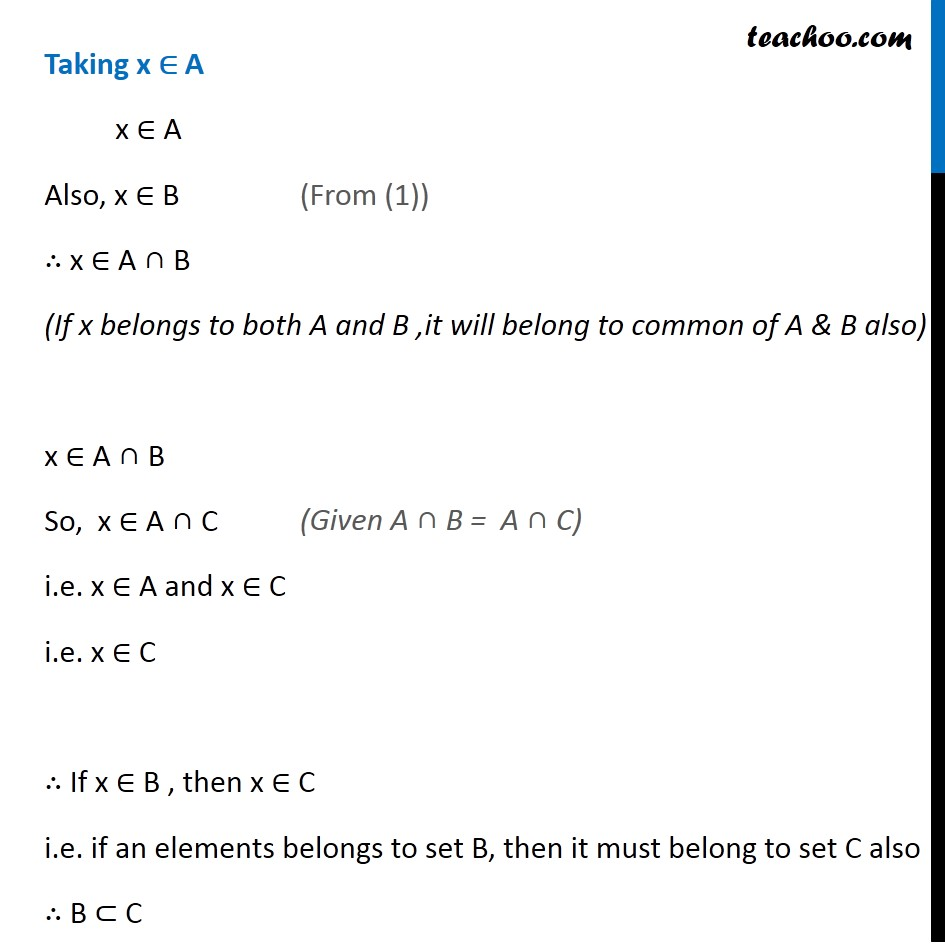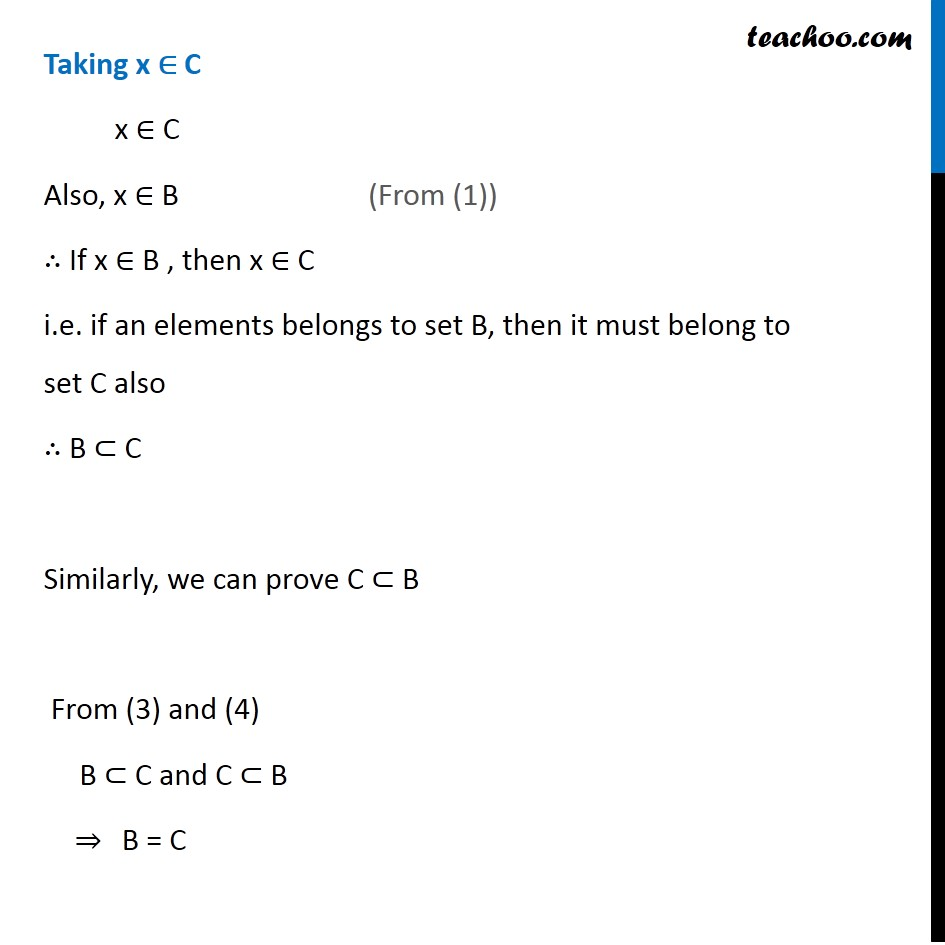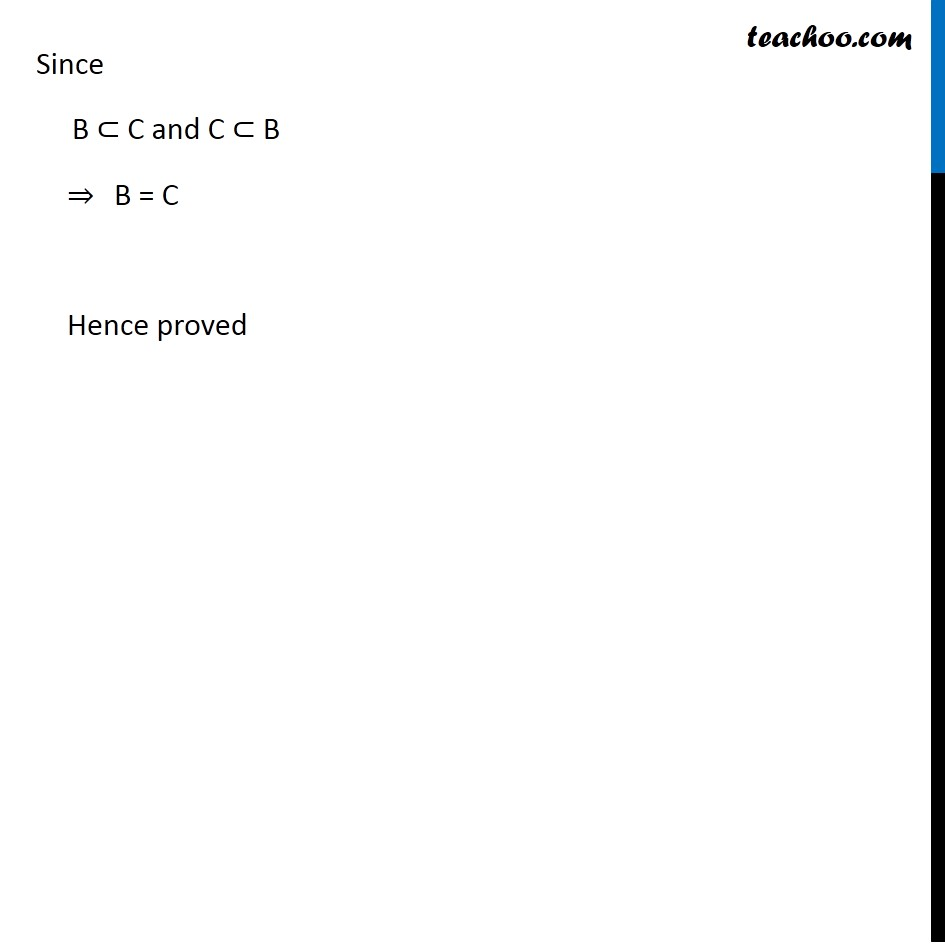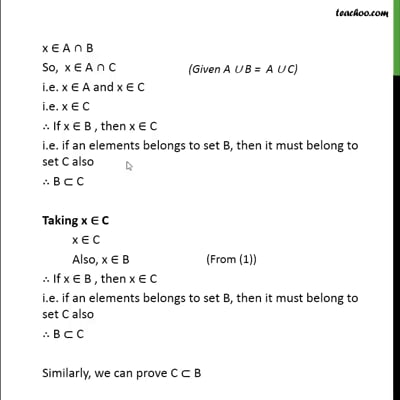This video is only available for Teachoo black users

### Transcript

Misc 3 Let A, B and C be the sets such that A ∪ B = A ∪ C and A ∩ B = A ∩ C. show that B = C. In order to prove B = C, we should prove B is a subset of C i.e. B ⊂ C & C is a subset of B i.e. C ⊂ B Let x ∈ B ⇒ x ∈ A ∪ B ⇒ x ∈ A ∪ C ⇒ x ∈ A or x ∈ C (Since B ⊂ A ∪ B, all elements of B are in A ∪ B) (Given A ∪ B = A ∪ C) Taking x ∈ A x ∈ A Also, x ∈ B ∴ x ∈ A ∩ B (If x belongs to both A and B ,it will belong to common of A & B also) x ∈ A ∩ B So, x ∈ A ∩ C i.e. x ∈ A and x ∈ C i.e. x ∈ C ∴ If x ∈ B , then x ∈ C i.e. if an elements belongs to set B, then it must belong to set C also ∴ B ⊂ C (From (1)) (Given A ∩ B = A ∩ C) Taking x ∈ C x ∈ C Also, x ∈ B ∴ If x ∈ B , then x ∈ C i.e. if an elements belongs to set B, then it must belong to set C also ∴ B ⊂ C Similarly, we can prove C ⊂ B From (3) and (4) B ⊂ C and C ⊂ B ⇒ B = C (From (1)) Since B ⊂ C and C ⊂ B ⇒ B = C Hence proved